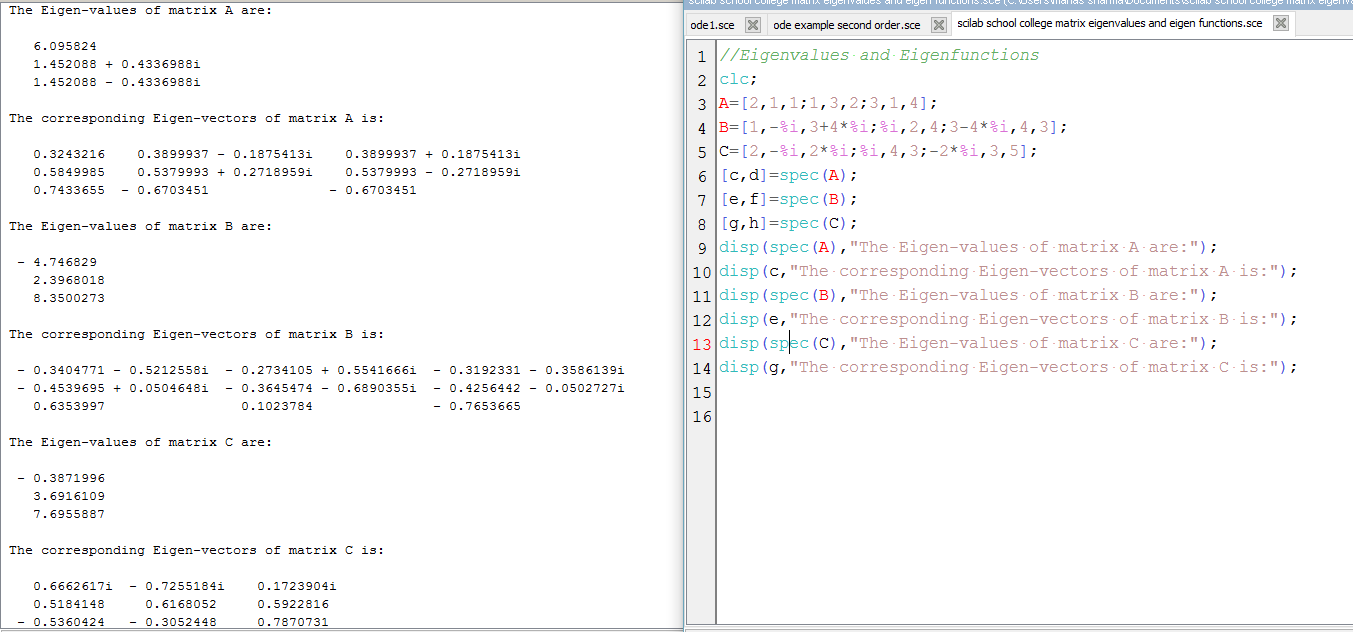# Eigenvalues and Eigenvectors in SCILAB

Scilab has an inbuilt function called spec(A) to calculate the Eigenvalues of a Matrix A.
If you type,
[c,d]=spec(A)
where d is a diagonal matrix which contains the eigen-values,
and c is a matrix that stores the eigen-vectors as it’s columns.

The following code illustrates the use of the function spec(A) to print the eigenvalues and eigenvectors of Matrices.

```//Eigenvalues and Eigenfunctions
clc;
A=[2,1,1;1,3,2;3,1,4];
B=[1,-%i,3+4*%i;%i,2,4;3-4*%i,4,3];
C=[2,-%i,2*%i;%i,4,3;-2*%i,3,5];
[c,d]=spec(A);
[e,f]=spec(B);
[g,h]=spec(C);
disp(spec(A),"The Eigen-values of matrix A are:");
disp(c,"The corresponding Eigen-vectors of matrix A is:");
disp(spec(B),"The Eigen-values of matrix B are:");
disp(e,"The corresponding Eigen-vectors of matrix B is:");
disp(spec(C),"The Eigen-values of matrix C are:");
disp(g,"The corresponding Eigen-vectors of matrix C is:");```

Output:Video:[wpedon id="7041" align="center"]

## 2 thoughts on “Eigenvalues and Eigenvectors in SCILAB”

1. Dear Sir,
I am a Ph.D. student and working on DFT. I want to learn Siesta but there are very limited materials I get from google. Please make some videos on Siesta.